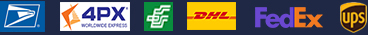•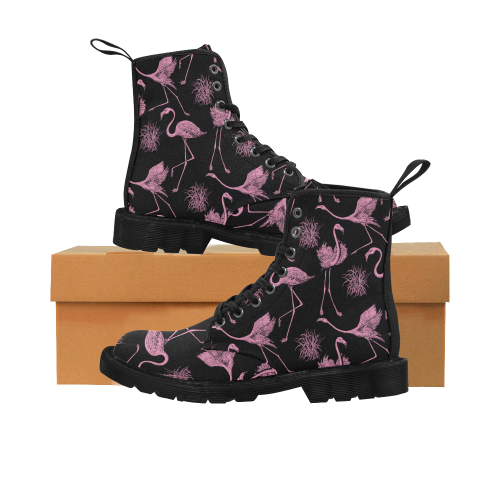•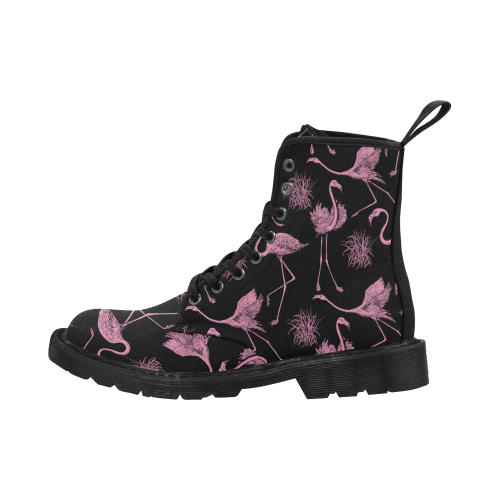•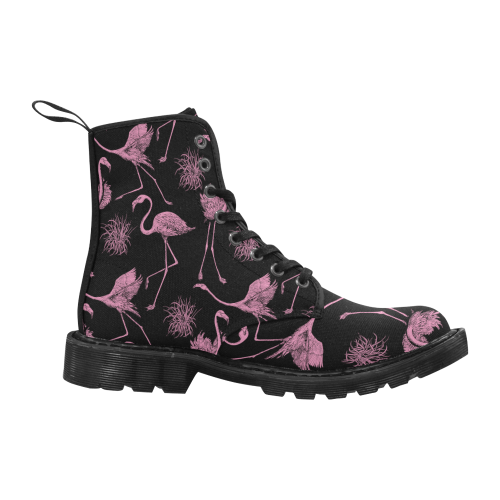•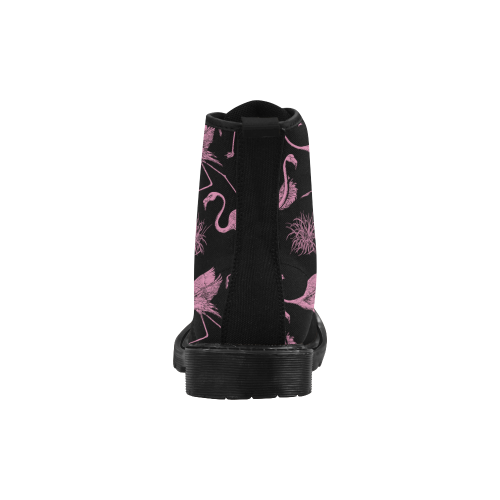•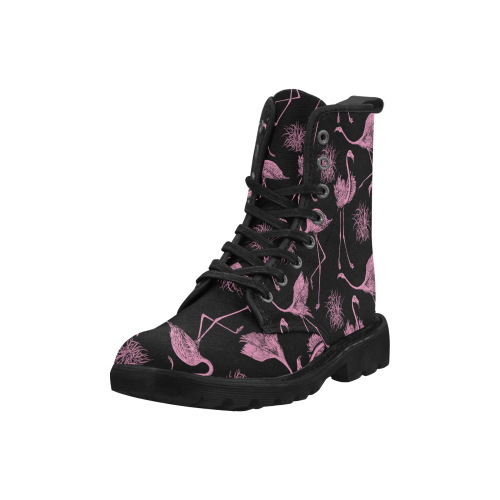•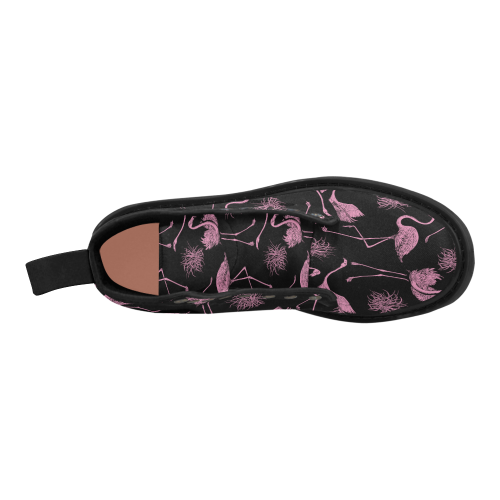### Women's Lace Up Canvas Boots (Model1203H)(Black)

\$39.99
Retail Price \$69.99

Shipping Included

•   Black
EUR36 = US6.5 =UK4=236mm =9.29inch
EUR37 = US7=UK4.5 = 243mm = 9.57inch
EUR38 = US7.5 =UK5.5= 250mm = 9.84inch
EUR39 = US8.5 =UK6= 256mm = 10.08inch
EUR40 = US9 =UK6.5= 261mm = 10.28inch

EUR41 = US9.5 =UK7= 263mm = 10.35inch

EUR42 = US10 =UK7.5= 268mm = 10.55inch

EUR43 = US11 =UK8 = 275mm = 10.83inch

EUR44 = US12 =UK8.5 = 281mm = 11.18inch

Payment methods we accpet: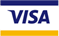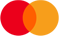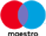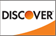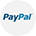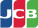We deliver with: